• 您的当前位置： 运营干货> 运营方案>正文

4S店8月营销方案+模板出炉！还有淡季店头龙虾美食节活动实战案例

• 发布时间：2019-08-08
• 标签:4S店营销方案，4S店活动软文，4S店销售活动

我们在前几天举办了新品发布会

会上宣布

车商通品牌正式升级为“知店”

原“车商通”则为子产品

同时发布了两款

汽车行业数字化转型革命性产品

知店"全民经纪人"和“智能客流

解决车商自获客、渠道下沉和客流管理难题

言归正传

本期方案除了常规

【8月营销方案】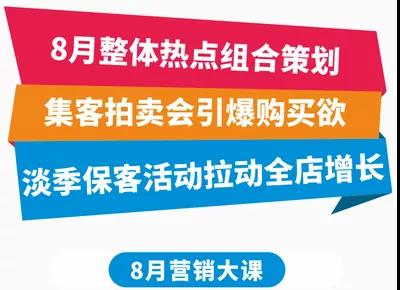还给大家带来了

【淡季店头龙虾美食节活动实战案例】课程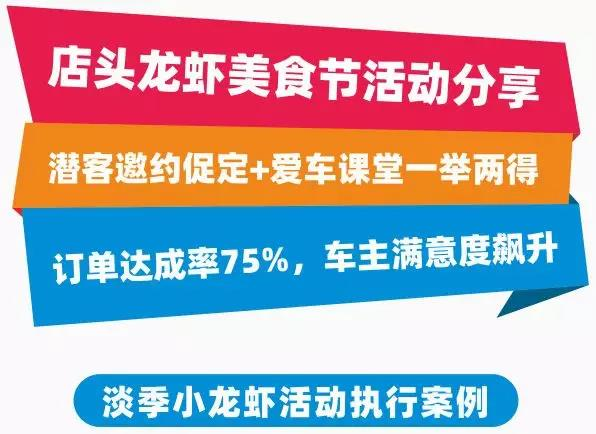下面开始介绍8月

素材模板+活动方案

七夕节素材模板推荐七夕让她心跳加速的秘籍？这里有图解！

使用场景：销售促销

创作解析：通过最近热映的电视剧《亲爱的热爱的》里面的浪漫桥段做比喻，最后引申到“为了你，我想买辆车”，从而带出文末的店头团购促销活动恋爱中的女人都是福尔摩斯！知识点速学！

使用场景：店头活动

创作解析：通过描述女人在恋爱中强大的第六感，吸引客户阅读，从而带出店内的七夕活动，帮客户制造惊喜，刺激客户报名到店七夕主题风格通用排版

使用场景：促销团购

创作解析：纯粹的通用排版，可搭配店内任意促销活动宣传

早安海报系列

7种风格7天任意切换

7×7=49种组合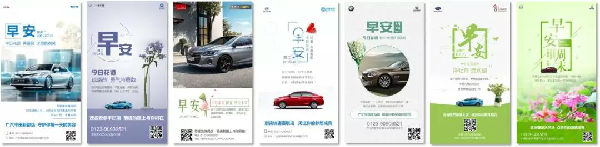促销团购系列素材推荐19年过了一半还没买车？这次的清库/团购来看看？

使用场景：促销团购

创作解析：通过突出2019已过一半，提醒客户把买车列入日程，简单直接切入店头促销团购活动意难平？大促返场，新增限量名额，别再错过

使用场景：促销团购

创作解析：简单粗暴的店头促销团购活动宣传，排版清晰，层次分明，刺激客户参与

促销团购海报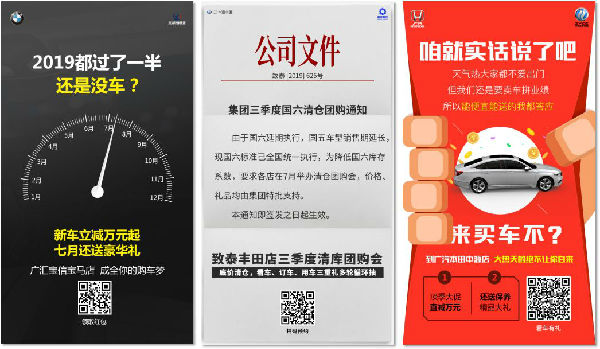活动即将开启预热气氛渲染

车商通倒计时海报系列

海报+头像一站式搞定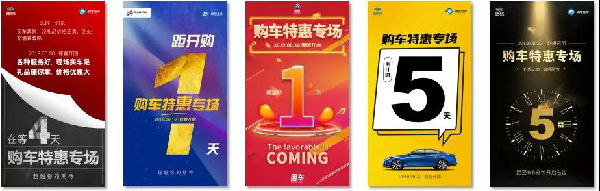电脑输入pc.chexiu.cn

登录车商通后台

即可免费使用全部软文模板海报模板以及

获取8月营销方案

淡季店头龙虾美食节活动实战案例PPT随后附上

车商通美女培训师

在线讲解8月营销活动思路

在线讲解淡季店头龙虾美食节活动实战案例

我们下个月不见不散

每月准时为您提供营销热点方案

4S店伙伴一定要持续关注车商通哦！

• 热门阅读X
• 在线咨询
• 400-8008-219
•• 友情链接：

• 请选择客户类型
客户类型
• 4S店
• 主机厂
• 4S集团
• 其他
• 品牌
所在城市
• 公司全称
• 请选择您的职位
职位
• 董事长
• 总经理
• 副总经理/总监
• 服务经理
• 客服经理
• 销售经理
• 销售顾问
• 市场经理
• 市场专员
• 网销主管
• IT部
• 其他
• 姓名
• 手机
• 图形码
• 获取验证码
验证码
• 收不到短信？您可 语音获取
车商通将拨打您的手机告知动态码
通讯异常，请重试
• 账号不能为空

• ABCDE
• FGHIJ
• KLMNO
• PQRST
• UVWXYZ
• A
• 爱驰汽车
• ALPINA
• 安凯客车
• 阿尔法罗密欧
• 北汽新能源
• 阿斯顿·马丁
• 一汽-大众奥迪
• 奥迪(进口)
• 平行进口奥迪
• Audi Sport
• 艾康尼克ICONIQ Motors
• B
• 巴博斯
• 平行进口巴博斯
• 拜腾
• 上汽通用别克
• 进口别克
• 宾利
• 平行进口宾利
• 布加迪
• 北汽昌河
• 北汽昌河新能源
• 北京汽车制造厂
• 北汽幻速
• 北汽新能源
• 上汽通用五菱
• 上汽通用五菱新能源
• 比速汽车
• 宝沃汽车
• 华晨宝马
• 宝马(进口)
• 宝马M
• 平行进口宝马
• 保时捷
• 平行进口保时捷
• 平行进口彼得比尔特
• 北京越野
• 博郡汽车
• 福建奔驰
• 北京奔驰
• 奔驰(进口)
• 平行进口奔驰
• 梅赛德斯-AMG
• 梅赛德斯-迈巴赫
• 梅赛德斯-EQ
• 一汽奔腾
• 东风本田
• 广汽本田
• 进口本田
• 平行进口本田
• 北京汽车
• 北汽瑞丽
• 星驰汽车
• 北汽威旺新能源
• 北汽威旺
• 比亚迪
• 东风标致
• 进口标致
• C
• 长安汽车
• 长安汽车新能源
• 长城汽车
• 长安轻型车
• 长安汽车
• 成功汽车
• 长江汽车
• 长安跨越
• 长安跨越新能源
• D
• 东风新能源
• 东风风度
• 进口大发
• 上汽大众
• 一汽-大众
• 大众(进口)
• 平行进口大众
• 雪铁龙(进口)
• 长安标致雪铁龙
• 东南道奇
• 道奇(进口)
• 平行进口道奇
• 东风汽车
• 比克汽车
• 东风时空
• 郑州日产
• 郑州日产新能源
• 东风小康
• 东风乘用车
• 东风风光
• 东南汽车
• 电咖汽车
• 东风风行
• 大运汽车
• 大乘汽车
• F
• 福汽新龙马
• 福迪汽车
• 法拉利
• 平行进口法拉利
• 福莱纳房车
• 菲亚特(进口)
• 广汽菲克菲亚特
• 南京菲亚特
• 广汽丰田
• 一汽丰田
• 丰田(进口)
• 平行进口丰田
• 长安福特
• 福特(进口)
• 江铃福特
• 平行进口福特
• 福田汽车
• 福田商用车
• G
• 国金汽车
• 广汽吉奥
• 国机智骏
• 广汽新能源
• 广汽丰田新能源
• 广汽本田新能源
• 广汽三菱新能源
• 光冈汽车
• 观致汽车
• GMC
• 平行进口GMC
• 广汽乘用车
• H
• 华人运通
• 广汽蔚来
• HOFELE
• 华晨华颂
• 长城汽车
• 东风悦达起亚
• 汉龙汽车
• 红星汽车
• 汉腾汽车
• Hennessey
• 恒天汽车
• 苏州金龙
• 哈飞汽车
• 一汽海马
• 海马汽车
• 悍马
• 平行进口悍马
• 一汽红旗
• 华普汽车
• 华泰汽车
• 华泰新能源
• 曙光汽车
• J
• 吉利汽车
• 吉利新能源
• 奇点汽车
• Jeep(进口)
• 广汽菲克Jeep
• SRT
• 平行进口Jeep
• 江铃汽车
• 江淮汽车
• 江淮新能源
• 江淮轻卡
• 捷豹
• 奇瑞捷豹路虎
• 华晨雷诺
• 绵阳金杯
• 华晨鑫源
• 君马汽车
• 江铃集团新能源
• 九龙汽车
• 厦门金旅
• 钧天汽车
• 厦门金龙
• 几何汽车
• 一汽-大众捷达
• 奇瑞汽车
• K
• KTM
• 开瑞汽车
• 通用凯迪拉克
• 凯迪拉克(进口)
• 平行进口凯迪拉克
• 凯翼汽车
• 科尼赛克
• 北京克莱斯勒
• 东南克莱斯勒
• 进口克莱斯勒
• 开沃汽车
• 卡威汽车
• 凯伦宾威
• 卡尔森
• L
• 领途汽车
• 一汽丰田朗世
• Lorinser
• 猎豹汽车
• 猎豹汽车新能源
• LOCAL MOTORS
• 兰博基尼
• 劳斯莱斯
• 雷克萨斯
• 平行进口雷克萨斯
• 雷诺(进口)
• 东风雷诺
• 领克
• 力帆汽车
• 路特斯
• 雷丁
• 莲花汽车
• 林肯
• 长安林肯
• 平行进口林肯
• 长安铃木
• 昌河铃木
• 进口铃木
• 陆风汽车
• 路虎(进口)
• 奇瑞捷豹路虎
• 平行进口路虎
• 零跑汽车
• 理想
• 罗夫哈特
• 广汽理念
• M
• 明君汽车
• Mansory
• 平行进口迈凯伦
• 迈凯伦
• 摩根
• 上汽名爵
• 长安马自达
• 一汽马自达
• 马自达(进口)
• 玛莎拉蒂
• 平行进口玛莎拉蒂
• 迈巴赫
• 平行进口迈巴赫
• MINI
• MINI JCW
• O
• 长城新能源
• 一汽欧朗
• 讴歌(进口)
• 广汽讴歌
• 欧宝
• Q
• 前途汽车
• 乔治·巴顿
• 平行进口乔治·巴顿
• 康迪电动汽车集团
• 东风启辰
• 骐铃汽车
• 奇瑞汽车
• 奇瑞新能源
• 东风悦达起亚
• 进口起亚
• R
• 东风日产
• 郑州日产
• 日产(进口)
• 平行进口日产
• 上汽荣威
• 奇瑞瑞麒
• 如虎
• 瑞驰新能源
• S
• 东风本田新能源
• 华晨鑫源
• SRM鑫源新能源
• 精灵smart
• 萨博
• 东南三菱
• 进口三菱
• 平行进口三菱
• 北京三菱
• 广汽三菱
• 世爵
• 双环汽车
• 双龙汽车
• 斯巴鲁
• 上汽斯柯达
• 进口斯柯达
• 平行进口斯柯达
• 赛麟汽车
• 江淮大众
• 金康SERES
• 上汽大通
• SWM斯威汽车
• 陕汽重卡
• T
• 唐骏汽车
• 平行进口特斯拉
• 特斯拉中国
• 特斯拉（进口）
• 腾势汽车
• 天际汽车
• 泰卡特
• W
• 上汽通用五菱
• 威马汽车
• 潍柴汽车
• 沃克斯豪尔
• 奇瑞威麟
• 奇瑞万达客车
• 威兹曼
• 长安沃尔沃
• 沃尔沃(进口)
• 沃尔沃亚太
• 平行进口沃尔沃
• 长城汽车
• 蔚来
• 庆铃汽车
• 江西五十铃
• 五十铃
• X
• 新凯汽车
• 西雅特
• 北京现代
• 现代(进口)
• 四川现代
• 星途
• 上汽通用雪佛兰
• 雪佛兰(进口)
• 平行进口雪佛兰
• 新特汽车
• 东风雪铁龙
• 进口雪铁龙
• 小鹏汽车
• 上汽通用五菱
• Y
• 一汽凌源
• 南京依维柯
• 依维柯(进口)
• 野马汽车
• 云度新能源
• 游侠电车
• 裕路汽车
• 浙江永源
• 宇通客车
• 御捷新能源
• 吉利远程新能源
• 云雀汽车
• 天津一汽
• 一汽吉林
• 一汽红塔
• 一汽通用
• 江铃汽车
• 英菲尼迪(进口)
• 平行进口英菲尼迪
• 东风英菲尼迪
• 银隆新能源
• Z
• 华晨宝马
• 知豆电动车
• 中欧房车
• 镇江飞驰
• 华晨中华
• 中兴汽车
• 广汽中兴
• 众泰汽车
• 众泰新能源
• N
• 东风裕隆
• 合众汽车
• NEVS国能汽车
• P
• 帕加尼
• Polestar汽车